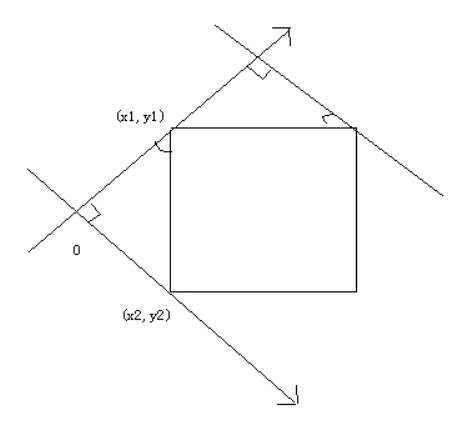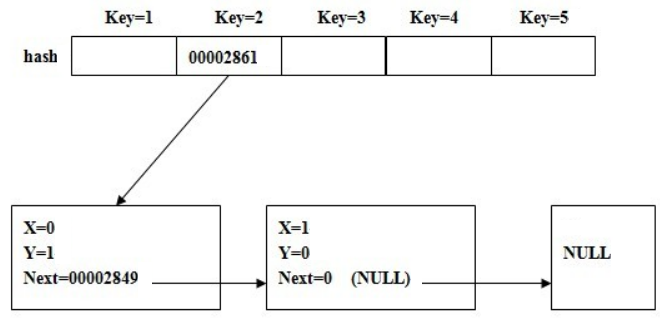# POJ 2002 - Squares

## 解题思路

``````x3=x1+(y1-y2)   y3= y1-(x1-x2)
x4=x2+(y1-y2)   y4= y2-(x1-x2)``````

``````x3=x1-(y1-y2)   y3= y1+(x1-x2)
x4=x2-(y1-y2)   y4= y2+(x1-x2)``````

• 当为 `k==1` 时，空间利用率最高，但地址冲突也相对较多，由于经常要为解决冲突开放寻址，使得寻找key值耗时O(1)的情况较少
• 当n太大时，空间利用率很低，但由于key分布很离散，地址冲突也相对较少，使得寻找键值耗时基本为O(1)的情况

``````K==1,   prime=997    1704ms
K==2,   prime=1999   1438ms
K==8,   prime=7993   1110ms
K==10,  prime=9973   1063ms
K==30,  prime=29989  1000ms
K==50,  prime=49999  1016ms
K==100, prime=99991  1000ms````````````typedef class HashTable
{
public:
int x,y;   // 标记key值对应的x,y

HashTable* next;  // 当出现地址冲突时，开放寻址

HashTable()  // Initial
{
next=0;
}

} Hashtable;

Hashtable* hash[prime];   // 注意hash[]是指针数组，存放地址

// hash[]初始化为NULL (C++初始化为0)``````

`key = (x^2+y^2)%prime+1`

`hash(x1，y1) = hash(x2，y2) = key`

• 当读入(x1, y1)时，若 `hash[key]` 为NULL，我们直接申请一个临时节点 `Hashtable* temp`，记录x1,y1的信息，然后把节点temp的地址存放到 `hash[key]` 中，此后我们就可以利用key访问temp的地址，继而得到x1,y1的信息
• 当读入(x2, y2)时，由于 `hash(x1，y1) = hash(x2，y2) = key` ，即(x2, y2)的信息同样要存入 `hash[key]`，但 `hash[key]` 已存有一个地址，怎么办？## AC 源码

``````//Memory Time
//652K  1438MS

#include<iostream>
using namespace std;

const int prime=1999;  //长度为2n区间的最大素数 (本题n=1000)

//其他prime可取值:
// 1n  区间: 997   1704ms
// 2n  区间: 1999  1438ms
// 8n  区间: 7993  1110ms
// 10n 区间: 9973  1063ms
// 30n 区间: 29989 1000ms
// 50n 区间: 49999 1016ms
// 100n区间: 99991 1000ms

//为了尽量达到key与地址的一一映射，hash[]至少为1n，
//当为1n时，空间利用率最高，但地址冲突也相对较多，由于经常要为解决冲突开放寻址，使得寻找key值耗时O(1)的情况较少
//当n太大时，空间利用率很低，但由于key分布很离散，地址冲突也相对较少，使得寻找键值耗时基本为O(1)的情况

typedef class
{
public:
int x,y;
}Node;

typedef class HashTable
{
public:
int x,y;   //标记key值对应的x,y
HashTable* next;  //当出现地址冲突时，开放寻址

HashTable()  //Initial
{
next=0;
}
}Hashtable;

Node pos;
Hashtable* hash[prime];   //hash[]是指针数组，存放地址

void insert_vist(int k)
{
int key=((pos[k].x * pos[k].x)+(pos[k].y * pos[k].y))%prime +1;   //+1是避免==0
//使key从[0~1998]后移到[1~1999]
if(!hash[key])
{
Hashtable* temp=new Hashtable;
temp->x=pos[k].x;
temp->y=pos[k].y;
hash[key]=temp;
}
else   //hash[key]已存地址，地址冲突
{
Hashtable* temp=hash[key];

while(temp->next)     //开放寻址，直至next为空
temp=temp->next;

temp->next=new HashTable;   //申请新节点，用next指向，记录x、y
temp->next->x=pos[k].x;
temp->next->y=pos[k].y;
}
return;
}

bool find(int x,int y)
{
int key=((x * x)+(y * y))%prime +1;

if(!hash[key])   //key对应的地址不存在
return false;
else
{
Hashtable* temp=hash[key];

while(temp)
{
if(temp->x==x && temp->y==y)
return true;

temp=temp->next;
}
}

return false;
}

int main(void)
{
int n;
while(cin>>n)
{
if(!n)
break;

memset(hash,0,sizeof(hash));   //0 <-> NULL

for(int k=1;k<=n;k++)
{
cin>>pos[k].x>>pos[k].y;
insert_vist(k);   //插入哈希表，标记散点
}

int num=0;  //正方形的个数
for(int i=1;i<=n-1;i++)
for(int j=i+1;j<=n;j++)
{
int a=pos[j].x-pos[i].x;
int b=pos[j].y-pos[i].y;

int x3=pos[i].x+b;
int y3=pos[i].y-a;
int x4=pos[j].x+b;
int y4=pos[j].y-a;

if(find(x3,y3) && find(x4,y4))
num++;

x3=pos[i].x-b;
y3=pos[i].y+a;
x4=pos[j].x-b;
y4=pos[j].y+a;

if(find(x3,y3) && find(x4,y4))
num++;
}

cout<<num/4<<endl;  //同一个正方形枚举了4次
}
return 0;
}``````

目录